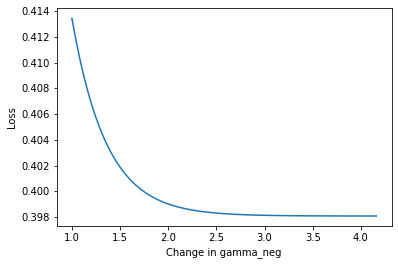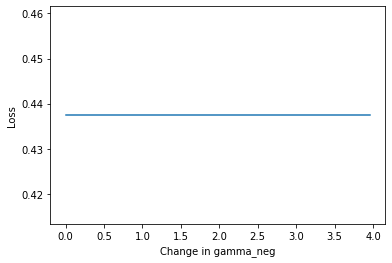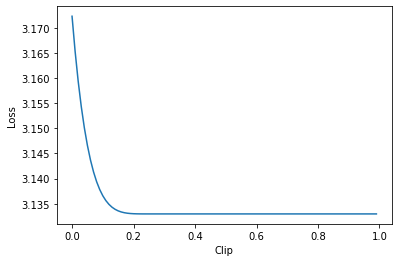This documentation is based on the paper "Asymmetric Loss For Multi-Label Classification".

## Asymetric Single-Label Loss

import timm
import torch
import torch.nn.functional as F
from timm.loss import AsymmetricLossMultiLabel, AsymmetricLossSingleLabel
import matplotlib.pyplot as plt
from PIL import Image
from pathlib import Path


Let's create a example of the output of a model, and our labels.

output = F.one_hot(torch.tensor([0,9,0])).float()
labels=torch.tensor([0,0,0])

labels, output

(tensor([0, 0, 0]),
tensor([[1., 0., 0., 0., 0., 0., 0., 0., 0., 0.],
[0., 0., 0., 0., 0., 0., 0., 0., 0., 1.],
[1., 0., 0., 0., 0., 0., 0., 0., 0., 0.]]))

If we set all the parameters to 0, the loss becomes F.cross_entropy loss.

asl = AsymmetricLossSingleLabel(gamma_pos=0,gamma_neg=0,eps=0.0)

asl(output,labels)

tensor(1.7945)
F.cross_entropy(output,labels)

tensor(1.7945)

Now lets look at the asymetric part. ASL is Asymetric in how it handles positive and negative examples. Positive examples being the labels that are present in the image, and negative examples being labels that are not present in the image. The idea being that an image has a lot of easy negative examples, few hard negative examples and very few positive examples. Getting rid of the influence of easy negative examples, should help emphasize the gradients of the positive examples.

Image.open(Path()/'images/cat.jpg')Notice this image contains a cat, that would be a positive label. This images does not contain a dog, elephant bear, giraffe, zebra, banana or many other of the labels found in the coco dataset, those would be negative examples. It is very easy to see that a giraffe is not in this image.

output = (2*F.one_hot(torch.tensor([0,9,0]))-1).float()
labels=torch.tensor([0,9,0])

losses=[AsymmetricLossSingleLabel(gamma_neg=i*0.04+1,eps=0.1,reduction='mean')(output,labels) for i in range(int(80))]

plt.plot([ i*0.04+1 for i,l in enumerate(losses)],[loss for loss in losses])
plt.ylabel('Loss')
plt.xlabel('Change in gamma_neg')
plt.show()$$L_- = (p)^{\gamma-}\log(1-p)$$

The contibution of small negative examples quickly decreases as gamma_neg is increased as $\gamma-$ is an exponent and $p$ should be a small number close to 0.

Below we set eps=0, this has the effect of completely flattening out the above graph, we are no longer applying label smoothing, so negative examples end up not contributing to the loss.

losses=[AsymmetricLossSingleLabel(gamma_neg=0+i*0.02,eps=0.0,reduction='mean')(output,labels) for i in range(100)]

plt.plot([ i*0.04 for i in range(len(losses))],[loss for loss in losses])
plt.ylabel('Loss')
plt.xlabel('Change in gamma_neg')
plt.show()## AsymmetricLossMultiLabel

AsymmetricLossMultiLabel allows for working on multi-label problems.

labels=F.one_hot(torch.LongTensor([0,0,0]),num_classes=10)+F.one_hot(torch.LongTensor([1,9,1]),num_classes=10)
labels

tensor([[1, 1, 0, 0, 0, 0, 0, 0, 0, 0],
[1, 0, 0, 0, 0, 0, 0, 0, 0, 1],
[1, 1, 0, 0, 0, 0, 0, 0, 0, 0]])
AsymmetricLossMultiLabel()(output,labels)

tensor(3.1466)

For AsymmetricLossMultiLabel another parameter exists called clip. This clamps smaller inputs to 0 for negative examples. This is called Asymmetric Probability Shifting.

losses=[AsymmetricLossMultiLabel(clip=i/100)(output,labels) for i in range(100)]

plt.plot([ i/100 for i in range(len(losses))],[loss for loss in losses])
plt.ylabel('Loss')
plt.xlabel('Clip')
plt.show()